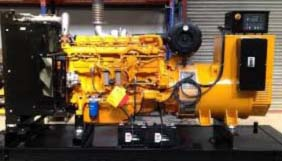Gas and Diesel Generator Maintenance Cost

# Gas and Diesel Generator Maintenance Cost

## Generator Maintenance

I would like to inform you the diesel generator maintenance cost , from the very beginning we found, our Generator has created too much high temperature. Some times Generator has shut off. For that reason, we informed your Engineers to solve this problem but they are unavailing to find out the problem and solve it. Several times our production has hampered. In front of your Engineers we have opened our Generator Heat Exchanger and we’ve found a big size (2.2”X2.2”) round metal pc. GETCO Engineer also agrees with us that this is the main problem for high temperature and also manufacturing fault.

### Generator Maintenance Proposal

With due respect would like to inform you that our Generator (GAS) have to servicing after every 1000 hours and also lube oil change. For this reason in next Friday January 21, 2010. from 01.00 pm. to 7.00 pm. want to set for servicing the Generator.  So, with beg most respectfully that you will give the permission for servicing the Generator on the above date and time.

### Materials/Spare Parts Rate For Gas Generator:

Life Time = 15 Years

= (15×12) Months

= 180 Months

### Generator Maintenance Cost

• Total Cost per Month for Generator = 18, 70,444 Tk.
• Total Cost per Year for Generator = (18, 70,444×12) Tk. = 2, 24, 45,328 Tk.
• Total Cost per Day for Generator = (2, 24, 45,328÷365) Tk. = 61,494 Tk.
• Total Cost per Hour for Generator = (61,494÷24) Tk. = 2,562 Tk.

### Generation Per Day (Average):

• Old Caterpillar Gas Generator = 12 MW
• New Caterpillar Gas Generator = 12 MW
• Total Generation per Day = (12+12) MW

= 24 MW

= (24×1,000) KW

= 24,000 KW

• Total Generation per Month = (24,000×28) KW

= 6, 72,000 KW

• Per KWH Cost = (18, 70,444÷6, 72,000) Tk.

= 2.78 Tk.

### Per Month Electricity Cost for All Units:

• Per Day electricity cost for Textile = (736.70×24) = 17,680.80 Tk.
• Per Month electricity cost for Textile = (17,680.80×28) = 4, 95,062.4 Tk.
• Per Day electricity cost for Generator Room = (108.42×24) = 2,602.08 Tk.
• Per Month electricity cost for Generator Room = (2602.08×28) = 72,858.24 Tk.
• Per Day electricity cost for Utility (Boiler Room) = (861.80×24) = 20,683.2 Tk.
• Per month electricity cost for Utility (Boiler Room) = (20,683.2×28) = 5, 79,129.6 Tk.
• Per Day electricity cost for Washing = (244.64×24) = 5871.36 Tk.
• Per Month electricity cost for Washing = (5871.36×28) = 1, 64,398.08 Tk.
• Per Day electricity cost for E.T.P-2 = (247.42×24) = 5,938.08 Tk.
• Per Month electricity cost for E.T.P-2 = (5,938.08×28) = 1, 66,266.24 Tk.
• Per Day electricity cost for Knitting = (264.10×24) = 6,338.4 Tk.
• Per Month electricity cost for Knitting = (6,338.4×28) = 1, 77,475.2 Tk.
• Per Day electricity cost for Garments = (1081.42×24) = 25,954.08 Tk.
• Per Month electricity cost for Garments = (25,954.08×28) = 7, 26,714.24 Tk.
• Per Day electricity cost for E.T.P-1 = (205.72×24) = 4,937.28 Tk.
• Per Month electricity cost for E.T.P-1 = (4,937.28×28) = 1, 38,243.84 Tk.
• Per Day electricity cost for Embroidery = (155.68×24) =3,736.32 Tk.
• Per Month electricity cost for Embroidery = (3,736.32×28) = 1, 04,616.96 Tk.

### Generator maintenance  – Cooling Tower

1. Ball Valve 1”
2. Butterfly Valve 4”(with Flange)
3. Butterfly Valve 6”(with Flange)
4. Butterfly Valve5”(with Flange)
5. Centrifuge High lift Pump
• Flow rate 120M/H
• Suction 6”
• Delivery 5”
1. Expunction Joint 4” (with Flange)
2. Expunction Joint 5”(with Flange)
3. Foot Valve 6”(Flange type, with Flange)
4. MS Elbow 2”
5. MS Elbow 4”
6. MS Elbow 5”
7. MS Elbow 6”
8. MS Reducer 4”*2”
9.  MS Reducer 4”*5”
10. MS Ring Flange 4”
11. MS Ring Flange 5”
12. MS Ring Flange 6”
13. MS Simless Pipe 4”
14. MS Simless Pipe 5”
15. MS Simless Pipe 6”
16. MS Simless Pipe 8”
17.  Non return Valve 5”(disk type)
18. Nut bolt with washer (full thread) 16*120
19. Nut bolt with washer 16*50
20. Nut bolt with washer 16*65
21. Nut bolt with washer 20*50
22. Nut bolt with washer 20*50
23. Pressure gauge
24. Strainer 6”       (Flange type, with Flange)
25. Temperature gauge (0-100,300)

### Generator maintenance – Exhaust Gas Line

1. MS Flange (as per sample)
2. MS Simless Pipe 12”
3. Temperature meter (0-500 c)

### Generator maintenance – Inlet Gas Line

1. Ball valve ½”
2. Ball Valve 4” (Flange type)
3. Gas Filter 4”(with Generator)
4. MS Elbow 4”
5. MS Flange 4”
6. MS Pipe 4”
7. MS Reducer ( 4”*3” welded type)
8. Niddle valve with sypone pipe ½”
9. Pressure gauge (0-200 PSI)
10. PRV (Gas regulator Valve3”)
11. S S Expansion Joint 4” (with flange)
12. Solenoid Valve 4” (with flange) with Generator